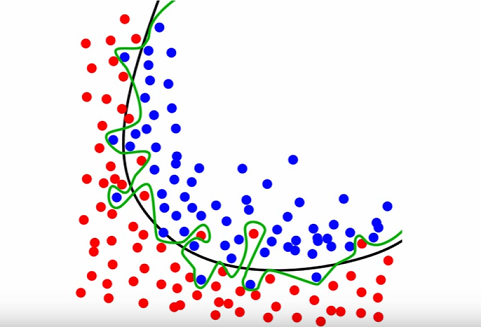## 2016年12月14日 星期三

### [ Scikit- learn ] Comparing machine learning models in scikit-learn

Source From Here
Preface

Agenda
* How do I choose which model to use for my supervised learning
* How do I choose the best turning parameters for that model
* How do I estimate the likely performance of my model on out-of-sample data

Review
* Classification task: Predicting the species of an unknown iris
* Used three classification models: KNN(K=1), KNN(K=5), logistic regression
* Need a way to choose between the models

Evaluation Procedure
Evaluation procedure #1: Train and test on the entire dataset

1. Train the model on the entire dataset
2. Test the model on the same dataset, and evaluate how well we did by comparing the predicted response values with the true response values

Logistic regression
>>> from sklearn.linear_model import LogisticRegression
>>> logreg = LogisticRegression() # Instantiate the model
>>> logreg.fit(X, y) # fit the model with data
LogisticRegression(C=1.0, class_weight=None, dual=False, fit_intercept=True,
intercept_scaling=1, max_iter=100, multi_class='ovr', n_jobs=1,
penalty='l2', random_state=None, solver='liblinear', tol=0.0001,
verbose=0, warm_start=False)

>>> y_pred = logreg.predict(X)
>>> len(y_pred)
150

Classification accuracy:
Proportion of correct predictions
* Common evaluation metric for classification problems
>>> from sklearn import metrics
>>> print metrics.accuracy_score(y, y_pred)
0.96

Known as training accuracy when you train and test the model on the same data

KNN(K=5)
>>> from sklearn.neighbors import KNeighborsClassifier
>>> knn = KNeighborsClassifier(n_neighbors=5)
>>> knn.fit(X, y)
KNeighborsClassifier(algorithm='auto', leaf_size=30, metric='minkowski',
metric_params=None, n_jobs=1, n_neighbors=5, p=2,
weights='uniform')

>>> print metrics.accuracy_score(y, knn.predict(X))
0.966666666667

KNN(K=1)
>>> knn = KNeighborsClassifier(n_neighbors=1)
>>> knn.fit(X, y)
KNeighborsClassifier(algorithm='auto', leaf_size=30, metric='minkowski',
metric_params=None, n_jobs=1, n_neighbors=1, p=2,
weights='uniform')

>>> print metrics.accuracy_score(y, knn.predict(X))
1.0 // Kind of overfitting issue!

Problems with training and testing on the same data
Can we draw the conclusion that the KNN(1) has the best performance? KNN(1) has already remember the training data for each training entry:
* Goal is to estimate likely performance of a model on out-of-sample data
* But, maximizing training accuracy rewards overly complex models that won't necessary generalize
* Unnecessarily complex models overfit the training dataGreen line impacted by noise and caused overfitting while the black line learn the signal and generally describe the training data set.

Evaluation procedure #2: Train/test split
1. Split the dataset into two pieces: a training set and a testing set
2. Train the model on the training set
3. Test the model on the testing set, and evaluate how well we did.
>>> from sklearn.cross_validation import train_test_split
>>> X_train, X_test, y_train, y_test = train_test_split(X, y, test_size=0.4, random_state=4)

What did this accomplish?
* Model can be trained and tested on different data (As out-of-sample)
* Response values are known for the training set, and thus predictions can be evaluated.
* Testing accuracy is a better estimate than training accuracy of out-of-sample performance.
>>> print X_train.shape
(90, 4)
>>> print X_test.shape

Logistic performance
>>> logreg.fit(X_train, y_train)
LogisticRegression(C=1.0, class_weight=None, dual=False, fit_intercept=True,
intercept_scaling=1, max_iter=100, multi_class='ovr', n_jobs=1,
penalty='l2', random_state=None, solver='liblinear', tol=0.0001,
verbose=0, warm_start=False)

>>> print metrics.accuracy_score(y_test, logreg.predict(X_test))
0.983333333333

KNN(K=1) Performance
>>> knn = KNeighborsClassifier(n_neighbors=1)
>>> knn.fit(X_train, y_train)
KNeighborsClassifier(algorithm='auto', leaf_size=30, metric='minkowski',
metric_params=None, n_jobs=1, n_neighbors=1, p=2,
weights='uniform')

>>> print "KNN(K=1) with performance=%.02f" % (metrics.accuracy_score(y_test, knn.predict(X_test)))
KNN(K=1) with performance=0.95

KNN(K=5) Performance
>>> knn = KNeighborsClassifier(n_neighbors=5)
>>> knn.fit(X_train, y_train)
KNeighborsClassifier(algorithm='auto', leaf_size=30, metric='minkowski',
metric_params=None, n_jobs=1, n_neighbors=5, p=2,
weights='uniform')

>>> print "KNN(K=1) with performance=%.02f" % (metrics.accuracy_score(y_test, knn.predict(X_test)))
KNN(K=1) with performance=0.97

* Training accuracy rises as model complexity increase
* Testing accuracy penalizes models that are too complex or not complex enough.
* For KNN models, complexity is determined by the value of K (lower value = more complex

Making predictions on out-of-sample data
1. try K=1 through K=25 and record testing accuracy
2. k_range = range(126)
3. score = []
4. for k in k_range:
5.     knn = KNeighborsClassifier(n_neighbors=k)
6.     knn.fit(X_train, y_train)
7.     y_pred = knn.predict(X_test)
8.     scores.append(metrics.accuracy_score(y_test, y_pred))
9.
10. import matplotlib.pyplot as plt
11.
12. # plot the relationship between K and testing accuracy
13. plt.plot(k_range, scores)
14. plt.xlabel('Value of K for KNN')
15. plt.ylabel('Testing Accuracy')

Supplement
Previous section - Training a machine learning model with scikit-learn
Next section - Data science in Python: pandas, seaborn, scikit-learn
* Quora explanation of overfitting: http://www.quora.com/What-is-an-intuitive-explanat...-overfitting/answer/Jessica-Su
* Estimating prediction error (video): https://www.youtube.com/watch?v=_2ij6eaaSl0&t=2m34s
* Understanding the Bias-Variance Tradeoff: http://scott.fortmann-roe.com/docs/BiasVariance.html
* Guiding questions for that article: https://github.com/justmarkham/DAT5/blob/master/homework/06_bias_variance.md
* Visualizing bias and variance (video): http://work.caltech.edu/library/081.html

### [ Python 文章收集 ] SQLAlchemy Core - Using Functions (7)

Source From   Here   Using Functions   Standard SQL has recommended many functions which are implemented by most dialects. They return a si...